Prealgebra

# 11.4Understand Slope of a Line

Prealgebra11.4 Understand Slope of a Line

### Learning Objectives

By the end of this section, you will be able to:
• Use geoboards to model slope
• Find the slope of a line from its graph
• Find the slope of horizontal and vertical lines
• Use the slope formula to find the slope of a line between two points
• Graph a line given a point and the slope
• Solve slope applications

### Be Prepared 11.4

Before you get started, take this readiness quiz.

1. Simplify: $1−48−2.1−48−2.$
If you missed this problem, review Example 4.49.
2. Divide: $04,40.04,40.$
If you missed this problem, review Example 7.37.
3. Simplify: $15−3,−153,−15−3.15−3,−153,−15−3.$
If you missed this problem, review Example 4.47.

As we’ve been graphing linear equations, we’ve seen that some lines slant up as they go from left to right and some lines slant down. Some lines are very steep and some lines are flatter. What determines whether a line slants up or down, and if its slant is steep or flat?

The steepness of the slant of a line is called the slope of the line. The concept of slope has many applications in the real world. The pitch of a roof and the grade of a highway or wheelchair ramp are just some examples in which you literally see slopes. And when you ride a bicycle, you feel the slope as you pump uphill or coast downhill.

### Use Geoboards to Model Slope

In this section, we will explore the concepts of slope.

Using rubber bands on a geoboard gives a concrete way to model lines on a coordinate grid. By stretching a rubber band between two pegs on a geoboard, we can discover how to find the slope of a line. And when you ride a bicycle, you feel the slope as you pump uphill or coast downhill.

### Manipulative Mathematics

Doing the Manipulative Mathematics activity "Exploring Slope" will help you develop a better understanding of the slope of a line.

We’ll start by stretching a rubber band between two pegs to make a line as shown in Figure 11.17.

Figure 11.17

Does it look like a line?

Now we stretch one part of the rubber band straight up from the left peg and around a third peg to make the sides of a right triangle as shown in Figure 11.18. We carefully make a $90°90°$ angle around the third peg, so that one side is vertical and the other is horizontal.

Figure 11.18

To find the slope of the line, we measure the distance along the vertical and horizontal legs of the triangle. The vertical distance is called the rise and the horizontal distance is called the run, as shown in Figure 11.19.

Figure 11.19

To help remember the terms, it may help to think of the images shown in Figure 11.20.

Figure 11.20

On our geoboard, the rise is $22$ units because the rubber band goes up $22$ spaces on the vertical leg. See Figure 11.21.

What is the run? Be sure to count the spaces between the pegs rather than the pegs themselves! The rubber band goes across $33$ spaces on the horizontal leg, so the run is $33$ units.

Figure 11.21

The slope of a line is the ratio of the rise to the run. So the slope of our line is $23.23.$ In mathematics, the slope is always represented by the letter $m.m.$

### Slope of a line

The slope of a line is $m=riserun.m=riserun.$

The rise measures the vertical change and the run measures the horizontal change.

What is the slope of the line on the geoboard in Figure 11.21?

$m=riserunm=riserun$
$m=23m=23$
$The line has slope23.The line has slope23.$

When we work with geoboards, it is a good idea to get in the habit of starting at a peg on the left and connecting to a peg to the right. Then we stretch the rubber band to form a right triangle.

If we start by going up the rise is positive, and if we stretch it down the rise is negative. We will count the run from left to right, just like you read this paragraph, so the run will be positive.

Since the slope formula has rise over run, it may be easier to always count out the rise first and then the run.

### Example 11.30

What is the slope of the line on the geoboard shown?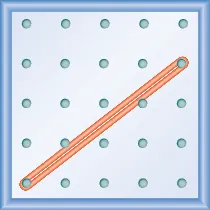### Try It 11.58

What is the slope of the line on the geoboard shown?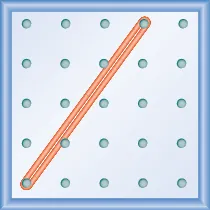### Try It 11.59

What is the slope of the line on the geoboard shown?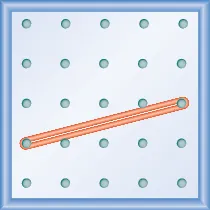### Example 11.31

What is the slope of the line on the geoboard shown?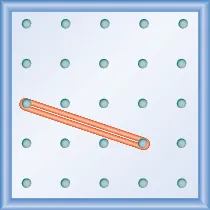### Try It 11.60

What is the slope of the line on the geoboard?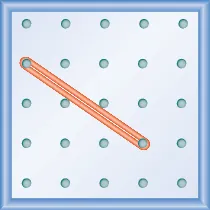### Try It 11.61

What is the slope of the line on the geoboard?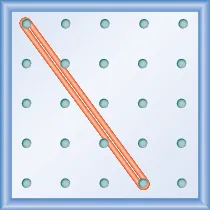Notice that in the first example, the slope is positive and in the second example the slope is negative. Do you notice any difference in the two lines shown in Figure 11.22.

Figure 11.22

As you read from left to right, the line in Figure A, is going up; it has positive slope. The line Figure B is going down; it has negative slope.

Figure 11.23

### Example 11.32

Use a geoboard to model a line with slope $12.12.$

### Try It 11.62

Use a geoboard to model a line with the given slope: $m=13.m=13.$

### Try It 11.63

Use a geoboard to model a line with the given slope: $m=32.m=32.$

### Example 11.33

Use a geoboard to model a line with slope $−14,−14,$

### Try It 11.64

Use a geoboard to model a line with the given slope: $m=−32.m=−32.$

### Try It 11.65

Use a geoboard to model a line with the given slope: $m=−13.m=−13.$

### Find the Slope of a Line from its Graph

Now we’ll look at some graphs on a coordinate grid to find their slopes. The method will be very similar to what we just modeled on our geoboards.

### Manipulative Mathematics

Doing the Manipulative Mathematics activity "Slope of Lines Between Two Points" will help you develop a better understanding of how to find the slope of a line from its graph.

To find the slope, we must count out the rise and the run. But where do we start?

We locate any two points on the line. We try to choose points with coordinates that are integers to make our calculations easier. We then start with the point on the left and sketch a right triangle, so we can count the rise and run.

### Example 11.34

Find the slope of the line shown: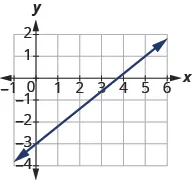### Try It 11.66

Find the slope of the line: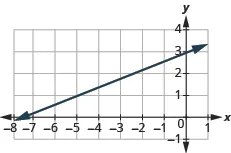### Try It 11.67

Find the slope of the line: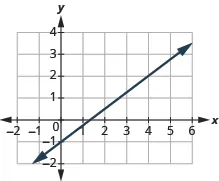### How To

#### Find the slope from a graph.

1. Step 1. Locate two points on the line whose coordinates are integers.
2. Step 2. Starting with the point on the left, sketch a right triangle, going from the first point to the second point.
3. Step 3. Count the rise and the run on the legs of the triangle.
4. Step 4. Take the ratio of rise to run to find the slope. $m=riserunm=riserun$

### Example 11.35

Find the slope of the line shown: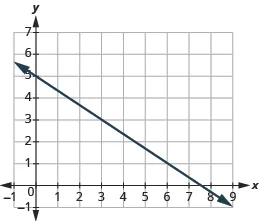### Try It 11.68

Find the slope of the line: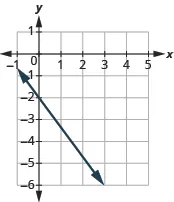### Try It 11.69

Find the slope of the line:The lines in the previous examples had $yy$-intercepts with integer values, so it was convenient to use the y-intercept as one of the points we used to find the slope. In the next example, the $yy$-intercept is a fraction. The calculations are easier if we use two points with integer coordinates.

### Example 11.36

Find the slope of the line shown: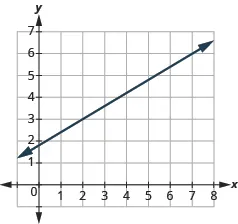### Try It 11.70

Find the slope of the line: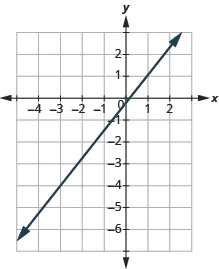### Try It 11.71

Find the slope of the line: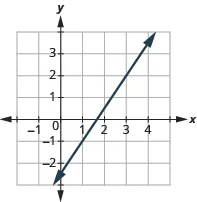### Find the Slope of Horizontal and Vertical Lines

Do you remember what was special about horizontal and vertical lines? Their equations had just one variable.

• horizontal line $y=b;y=b;$ all the $yy$-coordinates are the same.
• vertical line $x=a;x=a;$ all the $xx$-coordinates are the same.

So how do we find the slope of the horizontal line $y=4?y=4?$ One approach would be to graph the horizontal line, find two points on it, and count the rise and the run. Let’s see what happens in Figure 11.24. We’ll use the two points $(0,4)(0,4)$ and $(3,4)(3,4)$ to count the rise and run.

Figure 11.24
 What is the rise? The rise is 0. What is the run? The run is 3. What is the slope? $m=riserunm=riserun$ $m=03m=03$ $m=0m=0$

The slope of the horizontal line $y=4y=4$ is $0.0.$

All horizontal lines have slope $00$. When the $yy$-coordinates are the same, the rise is $00$.

### Slope of a Horizontal Line

The slope of a horizontal line, $y=b,y=b,$ is $0.0.$

Now we’ll consider a vertical line, such as the line $x=3x=3$, shown in Figure 11.25. We’ll use the two points $(3,0)(3,0)$ and $(3,2)(3,2)$ to count the rise and run.

Figure 11.25
 What is the rise? The rise is 2. What is the run? The run is 0. What is the slope? $m=riserunm=riserun$ $m=20m=20$

But we can’t divide by $0.0.$ Division by $00$ is undefined. So we say that the slope of the vertical line $x=3x=3$ is undefined. The slope of all vertical lines is undefined, because the run is $0.0.$

### Slope of a Vertical Line

The slope of a vertical line, $x=a,x=a,$ is undefined.

### Example 11.37

Find the slope of each line:

1. $x=8x=8$
2. $y=−5y=−5$

### Try It 11.72

Find the slope of the line: $x=−4.x=−4.$

### Try It 11.73

Find the slope of the line: $y=7.y=7.$

### Quick Guide to the Slopes of Lines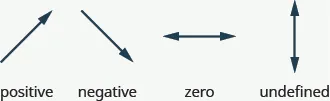### Use the Slope Formula to find the Slope of a Line between Two Points

Sometimes we need to find the slope of a line between two points and we might not have a graph to count out the rise and the run. We could plot the points on grid paper, then count out the rise and the run, but there is a way to find the slope without graphing.

Before we get to it, we need to introduce some new algebraic notation. We have seen that an ordered pair $(x,y)(x,y)$ gives the coordinates of a point. But when we work with slopes, we use two points. How can the same symbol $(x,y)(x,y)$ be used to represent two different points?

Mathematicians use subscripts to distinguish between the points. A subscript is a small number written to the right of, and a little lower than, a variable.

• $(x1,y1)readxsub1,ysub1(x1,y1)readxsub1,ysub1$
• $(x2,y2)readxsub2,ysub2(x2,y2)readxsub2,ysub2$

We will use $(x1,y1)(x1,y1)$ to identify the first point and $(x2,y2)(x2,y2)$ to identify the second point. If we had more than two points, we could use $(x3,y3),(x4,y4),(x3,y3),(x4,y4),$ and so on.

To see how the rise and run relate to the coordinates of the two points, let’s take another look at the slope of the line between the points $(2,3)(2,3)$ and $(7,6)(7,6)$ in Figure 11.26.

Figure 11.26

Since we have two points, we will use subscript notation.

$(2,3)x1,y1(7,6)x2,y2(2,3)x1,y1(7,6)x2,y2$

On the graph, we counted the rise of $3.3.$ The rise can also be found by subtracting the $y-coordinatesy-coordinates$ of the points.

$y2−y16−33y2−y16−33$

We counted a run of $5.5.$ The run can also be found by subtracting the $x-coordinates.x-coordinates.$

$x2−x17−25x2−x17−25$
 We know $m=riserunm=riserun$ So $m=35m=35$ We rewrite the rise and run by putting in the coordinates. $m=6−37−2m=6−37−2$ But 6 is the $yy$-coordinate of the second point, $y2y2$ and 3 is the $yy$-coordinate of the first point $y1y1$. So we can rewrite the rise using subscript notation. $m=y2−y17−2m=y2−y17−2$ Also 7 is the $xx$-coordinate of the second point, $x2x2$ and 2 is the $xx$-coordinate of the first point $x2x2$. So we rewrite the run using subscript notation. $m=y2−y1x2−x1m=y2−y1x2−x1$

We’ve shown that $m=y2−y1x2−x1m=y2−y1x2−x1$ is really another version of $m=riserun.m=riserun.$ We can use this formula to find the slope of a line when we have two points on the line.

### Slope Formula

The slope of the line between two points $(x1,y1)(x1,y1)$ and $(x2,y2)(x2,y2)$ is

$m=y2−y1x2−x1m=y2−y1x2−x1$

$Slope isyof the second point minusyof the first pointSlope isyof the second point minusyof the first point$
$overover$
$xof the second point minusxof the first point.xof the second point minusxof the first point.$

### Manipulative Mathematics

Doing the Manipulative Mathematics activity “Slope of Lines Between Two Points” will help you develop a better understanding of how to find the slope of a line between two points.

### Example 11.38

Find the slope of the line between the points $(1,2)(1,2)$ and $(4,5).(4,5).$

### Try It 11.74

Find the slope of the line through the given points: $(8,5)(8,5)$ and $(6,3).(6,3).$

### Try It 11.75

Find the slope of the line through the given points: $(1,5)(1,5)$ and $(5,9).(5,9).$

How do we know which point to call #1 and which to call #2? Let’s find the slope again, this time switching the names of the points to see what happens. Since we will now be counting the run from right to left, it will be negative.

 We’ll call $(4,5)(4,5)$ point #1 and $(1,2)(1,2)$ point #2. $(4,5)x1,y1and(1,2)x2,y2(4,5)x1,y1and(1,2)x2,y2$ Use the slope formula. $m=y2−y1x2−x1m=y2−y1x2−x1$ Substitute the values in the slope formula: $yy$ of the second point minus $yy$ of the first point $m=2−5x2−x1m=2−5x2−x1$ $xx$ of the second point minus $xx$ of the first point $m=2−51−4m=2−51−4$ Simplify the numerator and the denominator. $m=−3−3m=−3−3$ $m=1m=1$

The slope is the same no matter which order we use the points.

### Example 11.39

Find the slope of the line through the points $(−2,−3)(−2,−3)$ and $(−7,4).(−7,4).$

### Try It 11.76

Find the slope of the line through the pair of points: $(−3,4)(−3,4)$ and $(2,−1).(2,−1).$

### Try It 11.77

Find the slope of the line through the pair of points: $(−2,6)(−2,6)$ and $(−3,−4).(−3,−4).$

### Graph a Line Given a Point and the Slope

In this chapter, we graphed lines by plotting points, by using intercepts, and by recognizing horizontal and vertical lines.

Another method we can use to graph lines is the point-slope method. Sometimes, we will be given one point and the slope of the line, instead of its equation. When this happens, we use the definition of slope to draw the graph of the line.

### Example 11.40

Graph the line passing through the point $(1,−1)(1,−1)$ whose slope is $m=34.m=34.$

### Try It 11.78

Graph the line passing through the point with the given slope:

$(2,−2),m=43(2,−2),m=43$

### Try It 11.79

Graph the line passing through the point with the given slope:

$(−2,3),m=14(−2,3),m=14$

### How To

#### Graph a line given a point and a slope.

1. Step 1. Plot the given point.
2. Step 2. Use the slope formula to identify the rise and the run.
3. Step 3. Starting at the given point, count out the rise and run to mark the second point.
4. Step 4. Connect the points with a line.

### Example 11.41

Graph the line with $yy$-intercept $(0,2)(0,2)$ and slope $m=−23.m=−23.$

### Try It 11.80

Graph the line with the given intercept and slope:

$yy$-intercept $4,m=−524,m=−52$

### Try It 11.81

Graph the line with the given intercept and slope:

$xx$-intercept $−3,m=−34−3,m=−34$

### Example 11.42

Graph the line passing through the point $(−1,−3)(−1,−3)$ whose slope is $m=4.m=4.$

### Try It 11.82

Graph the line with the given intercept and slope: $(−2,1),m=3.(−2,1),m=3.$

### Try It 11.83

Graph the line with the given intercept and slope: $(4,−2),m=−2.(4,−2),m=−2.$

### Solve Slope Applications

At the beginning of this section, we said there are many applications of slope in the real world. Let’s look at a few now.

### Example 11.43

The pitch of a building’s roof is the slope of the roof. Knowing the pitch is important in climates where there is heavy snowfall. If the roof is too flat, the weight of the snow may cause it to collapse. What is the slope of the roof shown?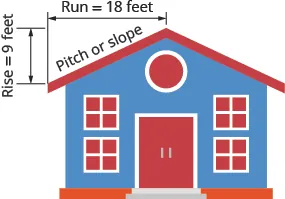### Try It 11.84

Find the slope given rise and run: A roof with a rise $=14=14$ and run $=24.=24.$

### Try It 11.85

Find the slope given rise and run: A roof with a rise $=15=15$ and run $=36.=36.$

Have you ever thought about the sewage pipes going from your house to the street? Their slope is an important factor in how they take waste away from your house.

### Example 11.44

Sewage pipes must slope down $1414$ inch per foot in order to drain properly. What is the required slope?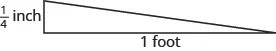### Try It 11.86

Find the slope of the pipe: The pipe slopes down $1313$ inch per foot.

### Try It 11.87

Find the slope of the pipe: The pipe slopes down $3434$ inch per yard.

### Section 11.4 Exercises

#### Practice Makes Perfect

Use Geoboards to Model Slope

In the following exercises, find the slope modeled on each geoboard.

203.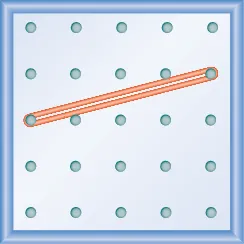204.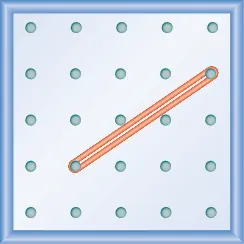205.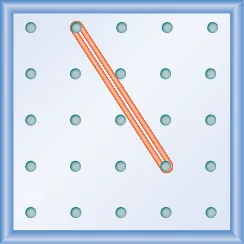206.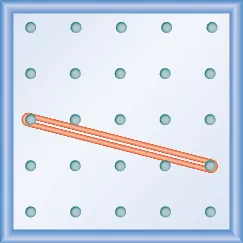In the following exercises, model each slope. Draw a picture to show your results.

207.

$2 3 2 3$

208.

$3 4 3 4$

209.

$1 4 1 4$

210.

$4 3 4 3$

211.

$− 1 2 − 1 2$

212.

$− 3 4 − 3 4$

213.

$− 2 3 − 2 3$

214.

$− 3 2 − 3 2$

Find the Slope of a Line from its Graph

In the following exercises, find the slope of each line shown.

215.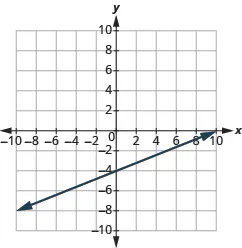216.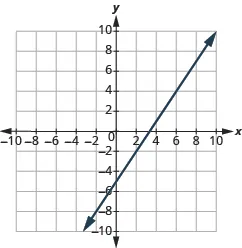217.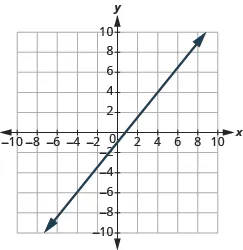218.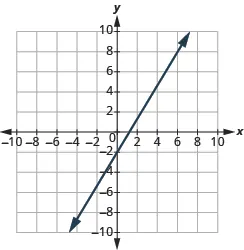219.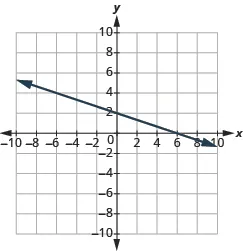220.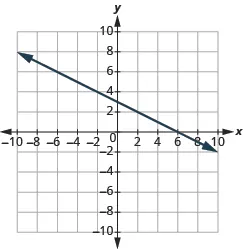221.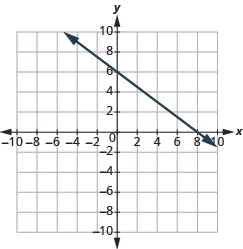222.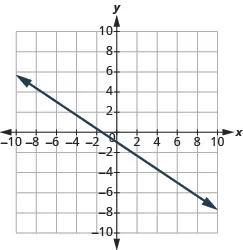223.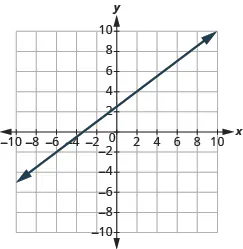224.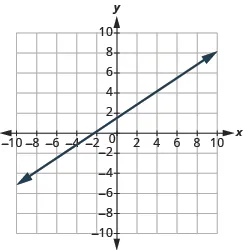225.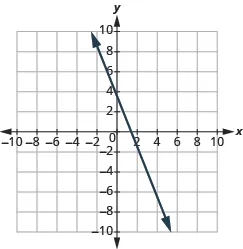226.227.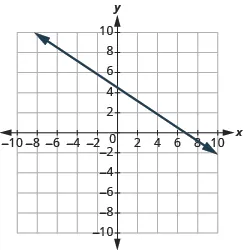228.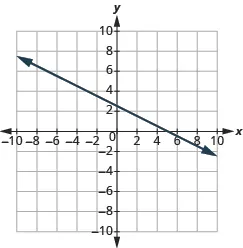229.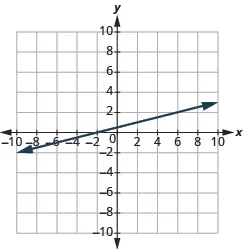230.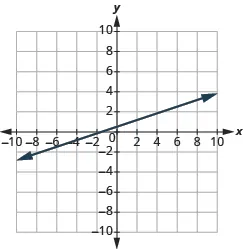Find the Slope of Horizontal and Vertical Lines

In the following exercises, find the slope of each line.

231.

$y = 3 y = 3$

232.

$y = 1 y = 1$

233.

$x = 4 x = 4$

234.

$x = 2 x = 2$

235.

$y = −2 y = −2$

236.

$y = −3 y = −3$

237.

$x = −5 x = −5$

238.

$x = −4 x = −4$

Use the Slope Formula to find the Slope of a Line between Two Points

In the following exercises, use the slope formula to find the slope of the line between each pair of points.

239.

$( 1 , 4 ) , ( 3 , 9 ) ( 1 , 4 ) , ( 3 , 9 )$

240.

$( 2 , 3 ) , ( 5 , 7 ) ( 2 , 3 ) , ( 5 , 7 )$

241.

$( 0 , 3 ) , ( 4 , 6 ) ( 0 , 3 ) , ( 4 , 6 )$

242.

$( 0 , 1 ) , ( 5 , 4 ) ( 0 , 1 ) , ( 5 , 4 )$

243.

$( 2 , 5 ) , ( 4 , 0 ) ( 2 , 5 ) , ( 4 , 0 )$

244.

$( 3 , 6 ) , ( 8 , 0 ) ( 3 , 6 ) , ( 8 , 0 )$

245.

$( −3 , 3 ) , ( 2 , −5 ) ( −3 , 3 ) , ( 2 , −5 )$

246.

$( −2 , 4 ) , ( 3 , −1 ) ( −2 , 4 ) , ( 3 , −1 )$

247.

$( −1 , −2 ) , ( 2 , 5 ) ( −1 , −2 ) , ( 2 , 5 )$

248.

$( −2 , −1 ) , ( 6 , 5 ) ( −2 , −1 ) , ( 6 , 5 )$

249.

$( 4 , −5 ) , ( 1 , −2 ) ( 4 , −5 ) , ( 1 , −2 )$

250.

$( 3 , −6 ) , ( 2 , −2 ) ( 3 , −6 ) , ( 2 , −2 )$

Graph a Line Given a Point and the Slope

In the following exercises, graph the line given a point and the slope.

251.

$( 1 , −2 ) ; m = 3 4 ( 1 , −2 ) ; m = 3 4$

252.

$( 1 , −1 ) ; m = 1 2 ( 1 , −1 ) ; m = 1 2$

253.

$( 2 , 5 ) ; m = − 1 3 ( 2 , 5 ) ; m = − 1 3$

254.

$( 1 , 4 ) ; m = − 1 2 ( 1 , 4 ) ; m = − 1 2$

255.

$( −3 , 4 ) ; m = − 3 2 ( −3 , 4 ) ; m = − 3 2$

256.

$( −2 , 5 ) ; m = − 5 4 ( −2 , 5 ) ; m = − 5 4$

257.

$( −1 , −4 ) ; m = 4 3 ( −1 , −4 ) ; m = 4 3$

258.

$( −3 , −5 ) ; m = 3 2 ( −3 , −5 ) ; m = 3 2$

259.

$( 0 , 3 ) ; m = − 2 5 ( 0 , 3 ) ; m = − 2 5$

260.

$( 0 , 5 ) ; m = − 4 3 ( 0 , 5 ) ; m = − 4 3$

261.

$( −2 , 0 ) ; m = 3 4 ( −2 , 0 ) ; m = 3 4$

262.

$( −1 , 0 ) ; m = 1 5 ( −1 , 0 ) ; m = 1 5$

263.

$( −3 , 3 ) ; m = 2 ( −3 , 3 ) ; m = 2$

264.

$( −4 , 2 ) ; m = 4 ( −4 , 2 ) ; m = 4$

265.

$( 1 , 5 ) ; m = −3 ( 1 , 5 ) ; m = −3$

266.

$( 2 , 3 ) ; m = −1 ( 2 , 3 ) ; m = −1$

Solve Slope Applications

In the following exercises, solve these slope applications.

267.

Slope of a roof A fairly easy way to determine the slope is to take a $12-inch12-inch$ level and set it on one end on the roof surface. Then take a tape measure or ruler, and measure from the other end of the level down to the roof surface. You can use these measurements to calculate the slope of the roof. What is the slope of the roof in this picture?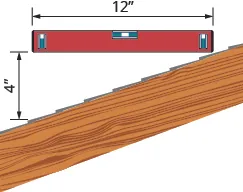268.

What is the slope of the roof shown?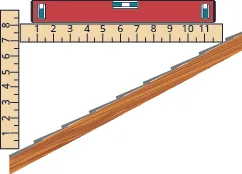269.

Road grade A local road has a grade of $6%.6%.$ The grade of a road is its slope expressed as a percent.

1. Find the slope of the road as a fraction and then simplify the fraction.
2. What rise and run would reflect this slope or grade?
270.

Highway grade A local road rises $22$ feet for every $5050$ feet of highway.

1. What is the slope of the highway?
2. The grade of a highway is its slope expressed as a percent. What is the grade of this highway?

#### Everyday Math

271.

Wheelchair ramp The rules for wheelchair ramps require a maximum $11$ inch rise for a $1212$ inch run.

1. How long must the ramp be to accommodate a $24-inch24-inch$ rise to the door?
2. Draw a model of this ramp.
272.

Wheelchair ramp A $1-inch1-inch$ rise for a $16-inch16-inch$ run makes it easier for the wheelchair rider to ascend the ramp.

1. How long must the ramp be to easily accommodate a $24-inch24-inch$ rise to the door?
2. Draw a model of this ramp.

#### Writing Exercises

273.

What does the sign of the slope tell you about a line?

274.

How does the graph of a line with slope $m=12m=12$ differ from the graph of a line with slope $m=2?m=2?$

275.

Why is the slope of a vertical line undefined?

276.

Explain how you can graph a line given a point and its slope.

#### Self Check

After completing the exercises, use this checklist to evaluate your mastery of the objectives of this section.

On a scale of 1–10, how would you rate your mastery of this section in light of your responses on the checklist? How can you improve this?

Order a print copy

As an Amazon Associate we earn from qualifying purchases.